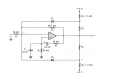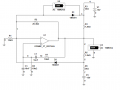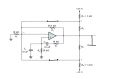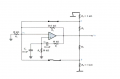# Opamp oscillator with amplitude stabilization

#### xxxyyyba

Joined Aug 7, 2012
289
It is known R4=R5=1.2k. My task is to calculate amplitude of oscillations at v0:Opamp and diodes are ideal.

I did analysis of circuit without amplitude stabilization and I got oscillation frequency, but I cant figure out how diodes stabilize amplitude in this circuit.
Any sugestion?

Edit: I simulated this circuit in Multisim:It looks like amplitude is 8.954*sqrt(2)V.
One strange thing: how can voltage on diode be 3.409V ??
I was thought that when diode is direct biased, voltage drop on it is about 0.7V.

Last edited:

#### Jony130

Joined Feb 17, 2009
5,457
All you need is to find Va and Vb voltage at point when D1 or D2 conducts. Also notice that Va node will swing up and down together with Vo.
For example:
D1 will be ON if Va = V1 - 0.7V = V1 if Vd = 0V and we can use a superposition to find Va
Va = 15V*R4/(R3+R4) + Vo' *R3/(R3 + R4)
So we have
V1 = 15V*R4/(R3+R4) + Vo' *R3/(R3 + R4)
And V1 is ??
One strange thing: how can voltage on diode be 3.409V ??
I was thought that when diode is direct biased, voltage drop on it is about 0.7V
The AC voltmeter show RMS value across the diode. So when diode is ON Vd = 0.7V but when diode is OFF the diode voltage is not zero.
And this is why RMS values is much larger than 0.7V.

•xxxyyyba

#### xxxyyyba

Joined Aug 7, 2012
289
The AC voltmeter show RMS value across the diode. So when diode is ON Vd = 0.7V but when diode is OFF the diode voltage is not zero.
And this is why RMS values is much larger than 0.7V.
It's clear now.

All you need is to find Va and Vb voltage at point when D1 or D2 conducts. Also notice that Va node will swing up and down together with Vo.
For example:
D1 will be ON if Va = V1 - 0.7V = V1 if Vd = 0V and we can use a superposition to find Va
Va = 15V*R4/(R3+R4) + Vo' *R3/(R3 + R4)
So we have
V1 = 15V*R4/(R3+R4) + Vo' *R3/(R3 + R4)
And V1 is ??
If I understand, I should analyse two circuits (assuming ideal diodes are conducting):
First with shorted vo:Second with shorted DC supplies:Right?

#### Jony130

Joined Feb 17, 2009
5,457
You do not need to use a superposition theorem. You can use any network analysis method you what.

#### xxxyyyba

Joined Aug 7, 2012
289
But when we have constant and periodic sources (v0 is sine wave), we analyse circuit using superposition theorem?

#### Jony130

Joined Feb 17, 2009
5,457
All our small-signal analysis is based on superpositions. Even your op-amp model is using linear approximation.

•xxxyyyba

#### Jony130

Joined Feb 17, 2009
5,457
Yes I neglected the current through diode because we want to find the turn-on point.
For example for Vo = 1Vpeak we have -3.57143V at point Vb node and 5V at Va node. And V1 = Vo * R1/(R2 + R1) ≈ Vo/3 ≈ 0.33Vpeak
So as you can see both diodes are OFF. So 1Vpeak is not our answer. The diode will be ON for the point where Vb = Vo/3.
And this is why we need to assume that the current through diodes is still negligible.
So we can write
(Vo - Vb)/R5 ≈ (Vb - Vee)/R6 and Vb = Vo/3 (turn on point).
All we need is to solve for Vo.

•xxxyyyba